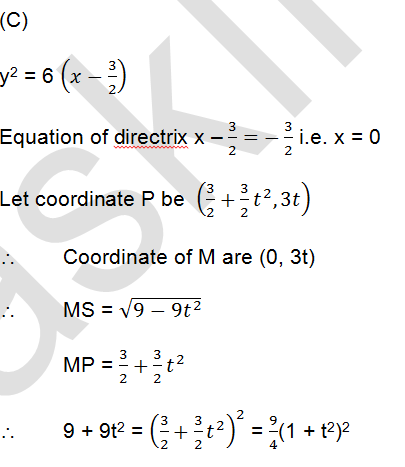# If P be a point on the parabola y2 = 3(2x – 3) and M is the foot of perpendicular drawn from P on the directrix of the parabola, then length of each side of an equilateral triangle SMP, where S is focus of the parabola, is            (a)       2            (b)       4            (c)        6            (d)       8

9 years ago4 = 1 + t2

Length of side = 6